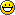Want to share your content on R-bloggers? click here if you have a blog, or here if you don't.

Earlier this year I came across a rather interesting page about optimisation in R from rwiki. The goal was to find the most efficient code to produce strings which follow the pattern below given a single integer input `n`:

```# n = 4
 "i001.002" "i001.003" "i001.004" "i002.003" "i002.004" "i003.004"
# n = 5
 "i001.002" "i001.003" "i001.004" "i001.005" "i002.003" "i002.004" "i002.005" "i003.004"
 "i003.005" "i004.005"
# n = 6
 "i001.002" "i001.003" "i001.004" "i001.005" "i001.006" "i002.003" "i002.004" "i002.005"
 "i002.006" "i003.004" "i003.005" "i003.006" "i004.005" "i004.006" "i005.006"
```

From this we can see that the general pattern for `n` is:

```# pseudo code
FOR j = 1 to n-1
FOR k = j+1 to n
PRINT "i" + format(j, digits = 3) + "." + format(k, digits = 3)
k = k + 1
END FOR
j = j + 1
END FOR
# this produces (n-1)*n/2 strings.
```

It is rather heart warming to go though that rwiki page and see how we can sequentially optimise the algorithm in R to more efficiently produce the desired string sequence. I learned quite a lot from this page about R and how fun these types of challenges can be!

Looking at the tenth solution, it achieves its speed by recognising that there are a total of n unique strings (e.g. “001″, “002″) to the pattern. These can be assigned to a character vector which we subsequently call by working out the sequence of indices we require and passing them to the character vector:

```generateIndex10 <- function(n) {
s <- sprintf("%03d", seq_len(n));
paste(
"i",
rep(s[1:(n-1)], (n-1):1),
".",
unlist(lapply(2:n, function(k) s[k:n]), use.names=FALSE),
sep=""
)
}
generateIndex10(7)
 "i001.002" "i001.003" "i001.004" "i001.005" "i001.006" "i001.007" "i002.003" "i002.004"
 "i002.005" "i002.006" "i002.007" "i003.004" "i003.005" "i003.006" "i003.007" "i004.005"
 "i004.006" "i004.007" "i005.006" "i005.007" "i006.007"
```

Playing around with the solution above, I noticed that a speed up would be possible if the following were implemented:

1. `rep` on a string vector is slower than simply using `rep.int` to work out the indices first and then passing those into the character vector.
2. Initialise the vectors to the correct size. This seems to produce a speed up though I’m not sure why it would in a vectorised solution. I could see the benefit if I were creating the vectors using an explicit R loop and concatenating the results but that is not the case here.
3. The functions could be compiled into bytecodes using the new compiler package (NB given that tenth solution is from 2007, this option would not have been available back then).
4. This problem could be set up to run in parallel. I didn’t do this because I’m still within running distance of being an R noobGiven the first three points above, this is the solution I came up with:

```generateIndex11 <- function(n) {
# initialise vectors
len <- (n-1)*n/2
s <- vector(mode = "character", length = n)
ind.1 <- vector(mode = "integer", length = len)
ind.2 <- vector(mode = "integer", length = len)

# set up strings
s <- sprintf("%03d", seq_len(n))

# calculate indices
ind.1 <- rep.int(1:(n-1), (n-1):1)
ind.2 <- unlist(lapply(2:n, ":", n), use.names = FALSE)

# paste strings together
return(paste("i", s[ind.1], ".", s[ind.2], sep = ""))
}
```

But is it faster? We can benchmark it and see!

```# load packages
library(compiler)
library(rbenchmark)

# compile functions
compiled_generateIndex10 <- cmpfun(generateIndex10)
compiled_generateIndex11 <- cmpfun(generateIndex11)

# set size
n = 5000

# run benchmark
benchmark(generateIndex10(n), compiled_generateIndex10(n), generateIndex11(n), compiled_generateIndex11(n),
columns = c("test", "replications", "elapsed", "relative"),
order = "relative",
replications = 10)

###--- TIMINGS ---###
test replications elapsed relative
4 compiled_generateIndex11(n)            10   51.46 1.000000
3          generateIndex11(n)            10   51.67 1.004081
2  compiled_generateIndex10(n)           10   54.35 1.056160
1           generateIndex10(n)           10   61.20 1.189273
```

So it seems that there is a speed up between `generateIndex10` and `generateIndex11`.   If we call the benchmark for different values of n and plot using the ggplot2 pack we get the following:

```library(ggplot2)
get_bm <- function(n) {
gc()
df = benchmark(generateIndex10(n), compiled_generateIndex10(n), generateIndex11(n), compiled_generateIndex11(n),
columns = c("test", "elapsed"),
order = "relative",
replications = 10)
df\$n = n
return(df)

}

df.list <- lapply(seq(1000, 9000, by = 250), get_bm)
df <- do.call("rbind", df.list)
ggplot(df, aes(x=n, y=elapsed, colour=test, shape=test)) + geom_point() + stat_smooth() + xlab("N") + ylab("Time (seconds)")
```

Which produces the graph below. I don’t know what’s happening at the extreme values but I suspect that it has something to do with memory because on my 8GB machine, the RAM was mostly at 99% for the largest values of n. I also like how stable the compiled versions of the functions are:In conclusion, whist this compiled eleventh solution is faster for larger values of `n`, for values below `1000` the tenth solution is sometimes faster.

Writing this post, I noticed something and actually came up with an even faster twelfth  solution. When I get time in the next couple of days I’ll make a post about it and also submit it to rwiki.

Thanks for reading, this was my first proper R blog post, constructive comments are most welcome! Also, please kindly bare in mind that I am not an R expert but rather just trying to improve my knowledge of the language